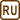## The Earth-Moon System

Determine the position of the centre of mass of the Earth-Moon system if it is known that the mass of the Earth is 81 times greater than the Moon's mass and the distance between their centres is 384 000 km. Compare the distance between the centre of the mass and the Earth's centre with the radius of the Earth.

• #### Notation

 m mass of the Moon M = 81m mass of the Earth a = 384 000 km distance between the centre of the Moon and centre of the Earth xm = ? distance between the centre of mass of the system and the centre of the Earth
• #### Hint 1

Draw the above-mentioned situation in an appropriate coordinate system. Which coordinates of the centre of mass of the system require calculation and which ones can be determined right from the figure without any calculation?

• #### Hint 2

In the given situation, we can consider Earth and Moon to be point masses because the distance between them (384 000 km) is many times greater than their dimensions (radius of the Earth is 6378 km). Therefore, in order to calculate the unknown coordinate xm, we can use the formula for the centre of mass of the system of point masses. How does this formula look like?

• #### Hint 3

Substitute the given values into relation (1) and calculate it. Express the distance between the centre of mass of the system and the centre of the Earth as a proportion of the Earth's radius.

• #### Overall Solution

First, we draw a picture of the situation (see below). The Earth and the Moon can be considered mass points because the distance between them (384 000 km) is many times greater than their dimensions (radius of the Earth is 6378 km). The coordinate system is chosen in a way that its origin lies in the centre of the Earth and its x-axis is passing through the centre of the Moon:Since the centres of mass of both considered bodies lie on the x-axis, centre of mass of their system will lie on the x-axis as well. All points of the x-axis have zero y-ovou and z-coordinates, which means that for the coordinates ym and zm of the centre of mass the following holds true:

$y_m\,=\,z_m\,=\,0.$

Therefore, in order to calculate the unknown coordinate xm, we can use the formula for the centre of mass of a system with n mass points:

$\vec{r}_m\,=\,\frac{\sum_{i=1}^n{m_i\vec{r}_i}}{\sum_{i=1}^n{m_i}},$

where mi is the mass of each mass point and ri is the associated position vector. Since the coordinates of the centre of mass can be calculated separately, for coordinate xm in our system we get:

$x_m\,=\,\frac{\sum_{i=1}^2{m_ix_i}}{\sum_{i=1}^2{m_i}}.\tag{1}$

After substitution into relation (1), we obtain:

$x_m\,=\,\frac{M{\cdot}0\,+\,m{\cdot}a}{M\,+\,m}\,=\,\frac{ma}{81m\,+\,m}\,=\,\frac{a}{82}.$

Numerically:

$x_m\,=\,\frac{384\,000}{82}\,\mathrm{km}\,\dot=\,4683\,\mathrm{km}.$

Since the radius of the Earth is 6378 km, distance xm makes approximately 73.4% of this value. Thus, the centre of mass of the Earth-Moon system is located below the Earth's surface (we can see the figure is not consistent with this fact).

$x_m\,\dot=\,4683\,\mathrm{km},$ $y_m\,=\,0\,\mathrm{km},$ $z_m\,=\,0\,\mathrm{km}.$